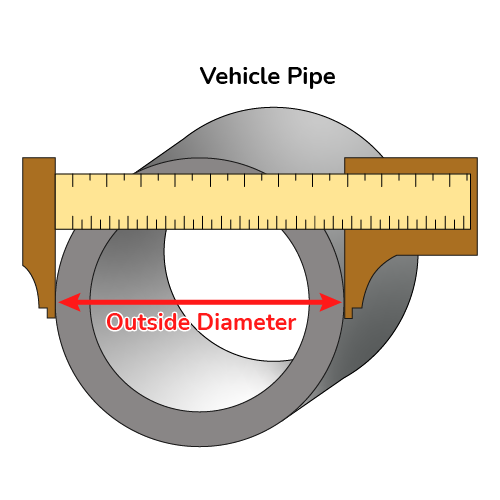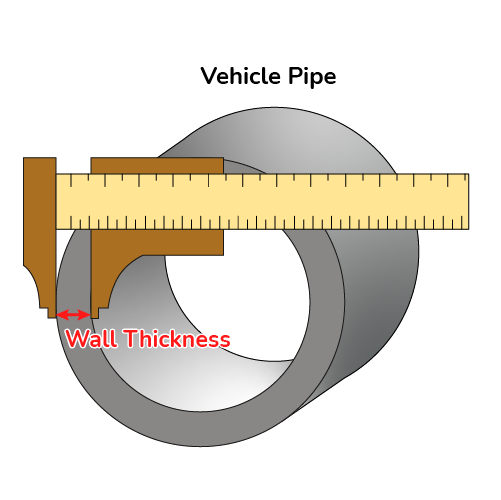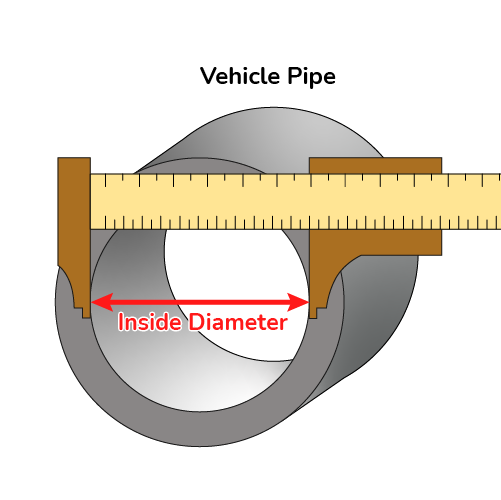# Exhaust Diameter Calculator

Created by Joanna Śmietańska, PhD candidate
Reviewed by Steven Wooding
Last updated: Jun 05, 2023

If you've ever wondered "what size exhaust pipe do I need for my automobile?", check out this exhaust diameter calculator. Choose the proper exhaust pipe sizes using our exhaust size calculator.

It allows you to determine exhaust diameter by knowing the engine's RPM and the volume of the vehicle's cylinder. It may seem that choosing an exhaust pipe size is a simple task and bigger always means better, but remember that matching the size of the exhaust pipe to the engine plays a key role in maximizing power at the low-end, mid-range, or top-end. Check our horsepower calculator to calculate your engine's power in horsepower.

If you're unsure how to measure an exhaust pipe, our exhaust diameter calculator will do it for you and calculate the diameter of the exhaust pipe based on the wall thickness and the outer diameter of the pipe. You will also learn what is a straight pipe exhaust and find the optimal exhaust diameter from the exhaust pipe size chart.

## Exhaust diameter formula

Exhaust diameter is measured as the internal diameter of an exhaust pipe of a car for optimal performance and exhaust outflow. During calculations, you need to consider the engine’s peak-torque RPM because exhaust diameter affects engine torque. You can easily find this value using RPM calculator.

Having your specific RPM at peak torque in mind, you want to select optimal exhaust pipe sizes. Since you have already determined the cross-sectional area of the tube, we can now calculate the exhaust pipe inside diameter using the formula:

$D = \sqrt{\frac{{\rm RPM}\times \pi \times V}{\text{88,200}}}$

where:

• $D$Exhaust diameter;
• $\rm RPM$ – Maximum rotations per minute, RPM at peak torque;
• $V$ – Single cylinder's volume; and
• $\text{88,200}$ – Mathematical constant.

You can use the engine's peak torque RPM to calculate the primary tube's cross-section area:

$\text{Cross-section} = \frac{V \times \rm RPM}{\text{88,200}}$

If you want to easily calculate a cylinder's volume on your own, check out the bore diameter and stroke of your engine. Then, use one of these formulas:

$\small{V = \pi \times \frac{\rm{bore}}{2}\times\frac{\rm{bore}}{2}\times \rm{stroke}}$

$\small{V = \rm{bore}\times \rm{bore}\times \rm{stroke}\times 0.7854}$

No matter which formula you choose, you should get the same result: the volume of a single cylinder in your engine.

If your engine has multiple cylinders, e.g., six, simply multiply your result by 6 to obtain total engine displacement. You can also use engine displacement calculator to find your engine's size.

## How to measure diameter of exhaust pipe?

You can calculate the exhaust pipe's internal diameter $\rm ID$ if you measure the tube's outer diameter and wall thickness. To calculate the inside diameter, you must subtract from the measured outer diameter $\rm OD$ the total wall thickness $d$ of the pipe (simply multiply wall thickness by 2) or use our exhaust size calculator:${\rm ID = OD} - (d \times 2)$## How to use exhaust diameter calculator?

You can quickly calculate the required exhaust pipe size in just a few steps:

1. Enter the RPM of your engine at maximum torque.

2. Enter the cylinder volume. You can look up this in your car's documentation or measure bore and stroke and then use our calculator. You can also enter the total displacement of the engine and the number of cylinders it has to find the volume of a single cylinder.

3. Calculate exhaust diameter using this exhaust size calculator.

If you would like to see how to measure an exhaust pipe, preferably with a caliper or micrometer, and calculate its diameter:

1. Determine the outer diameter of the exhaust pipe.
2. Measure the wall thickness.
3. The calculator with give you the internal diameter.

## What is a straight pipe exhaust?

A straight pipe exhaust is a system where the role of the exhaust pipe is played by a straight pipe. The exhaust pipe belongs to the exhaust system of your car. Just like a chimney in a house, it serves to release the exhaust gases from the vehicle into the air.

## FAQ

### What size exhaust do I need for a 1.8-liter car with a peak torque RPM of 6,500?

An exhaust diameter is 1.02 cm. This is calculated as follows. A 1.8-liter engine is equal to 1,800 cubic centimeters (cc), so a single cylinder's displacement will be 1.8 liter / 4 = 450 cc. You can find the exhaust diameter from the formula:

D = √(6,500 × 450 × 3.14159) / 88,200

The resulting exhaust diameter is 1.02 cm.

### How do I calculate cylinder's volume if I have several cylinders inside?

You may know the total engine displacement, but for the calculation, you will need the volume of a single cylinder. In this case:

1. Find the total volume of your engine.

2. Check the number of cylinders in your engine.

3. Divide the volume of your engine by the number of cylinders.

Cylinder's volume = displacement / number of cylinders

For example, a 2.0-liter four-cylinder engine has a volume of 0.5 liters, or 500 cc per cylinder.

### How do I measure diameter of exhaust pipe?

You should measure both the exhaust pipe's outer diameter and wall thickness. Try to use a caliper or micrometer rather than eye-balling with a tape measure. If the end of the pipe is blocked and you don't have any instrument handy, find the circumference of the pipe using a tailor's tape measure (or a piece of string, which you can then measure with a standard tape measure or ruler). Then divide the circumference by π (3.14) to get the outside diameter.

### What size exhaust pipe do I need?

Exhaust pipe size is connected with the engine's size and total displacement, so the higher the engine's output, the larger the pipes will be. Generally, you should match the exhaust diameter to the total engine displacement.

Check the exhaust pipe size chart below to see which exhaust pipe size will be suitable for the size and power of your engine.

🙋 Note that pipe size normally refers to the exhaust pipe's outer diameter.

Engine displacement (ci)

Power (hp)

Pipe diameter (in)

150-200

100-200

2.00-2.50

200-250

150-250

2.25-250

250-300

200-300

2.50-3.00

300-350

250-350

3.00-3.50

350-400

300-400

3.50

400-450

400-500

3.50-4.00

450-500

450

4.50

Joanna Śmietańska, PhD candidate
Engine parameters
Peak-torque RPM
Total engine displacement
cu in
Number of cylinders
Cylinder volume
cu in
Outside diameter
in
Wall thickness
in
Exhaust diameter
in
People also viewed…

### BMR - Harris-Benedict equation

Harris-Benedict calculator uses one of the three most popular BMR formulas. Knowing your BMR (basal metabolic weight) may help you make important decisions about your diet and lifestyle.

### Ideal egg boiling

Quantum physicist's take on boiling the perfect egg. Includes times for quarter and half-boiled eggs.

### Impact test

The impact test calculator determines the energy absorbed in Izod and Charpy impact tests.

### Wavenumber

Use the wavenumber calculator to determine the wavenumber from the wavelength or frequency and velocity of a wave.